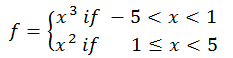Home MathLab Conditional Plotting in Matlab

# Conditional Plotting in Matlab

By now, you should have learned the basics of plotting in Matlab using previous post. In this session we want to look closer to how we can plot a conditional plot using Matlab Here is an easy example of a conditional plotplot f

## Conditional plotting in Matlab

One of the way you can walk to easily plot f is the following Matlab code

```x=-5:0.1:5;
for n=1:length(x)
if x(n)&lt;1
f(n)=x(n)^3;
else
f(n)=x(n)^2;
end
end
plot(x,f,'--r','linewidth',2)```

which returns

If you were just to plot half of the graph each time and add up plots, the code would look like

```% Part 1
x=-5:0.1:1;
f=x.^3;
plot(x,f,'--b','linewidth',2);
% Part 2
x=1:0.1:5;
f=x.^2;
hold on;
plot(x,f,'--r','linewidth',2)```

### Content you might like:

#### Related Posts

This website uses cookies to improve your experience. We'll assume you're ok with this, but you can opt-out if you wish. Accept Read More